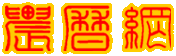共有58893人关注过本帖树形打印

# 主题：天文知识（专业） 一组宇宙计算公式原理凰帝 小大  1楼 个性首页 | 信息 | 搜索 | 邮箱 | 主页 | UC加好友发短信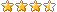等级：贵宾 帖子：218 积分：1329 威望：0 精华：5 注册：2005/9/4 16:47:00 天文知识（专业） 一组宇宙计算公式原理Post By：2005/9/16 20:12:00 您无权查看精华帖子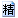[本帖被加为精华] 支持(2) 中立(0) 反对(1)2楼 个性首页 | 信息 | 搜索 | 邮箱 | 主页 | UC加好友发短信假定在宇宙中存在着许多小宇宙。由于某种原因，我们的这个小宇宙是个球状体，而且不断韵扩大。当它的半径达到临界距离 a0  时，就开始斥力膨胀，所以这个半径也叫临界半径或初半径。    由于类星体的数量，从红移Z=2.3以后,急剧减少,如图1所示，所以定义Z=2.3所对应的距离为这个小宇宙的最外层的视半径 a 。  S.Osmer, Ap.J.247, p762-773 (1981)3楼 个性首页 | 信息 | 搜索 | 邮箱 | 主页 | UC加好友发短信Matlab计算最大红移Zmax函数文件 1 ：zvr1.mfunction rv1=zv1(H)global Hrv1=1/0.003335641/H______________________________________________________________________函数文件 2 ： zerw.mfunction ze=zerw(r,M,a,V0)global M a V0k1=0.005296998;k2=0.240582497;k3=0.360873745;ze=(k1*V0*M*r*r+k2/r-k3*M/a)/(1-k1*V0*M*r*r-k2/r)______________________________________________________________________函数文件 3 ： zmax.mfunction zmax=zmax(r,H,M,a,V0)global H M a V0zmax=1.5+zerw(r)______________________________________________________________________M文件：  maxz.mglobal H M a V0H=input('H=');M=input('M=');a=input('a=');V0=input('V0=');zv1r=input('r=');zmax(r)4楼 个性首页 | 信息 | 搜索 | 邮箱 | 主页 | UC加好友发短信众所周知，星系的光学质量不能完全反映暗物质对星系质量的贡献。不过，暗物质的质量仍然要遵循经典力学。所以从道理上讲，维里质量*应当代表星系的质量。    另一方面，引力物质的特点是聚集。一般情况下；引力起控制作用的空间里，质量密度最大的地方一定是引力空间的中心区域，即天体及其周围。    根据上述看法，星系际不会有很多看不见的质量，超系团中也不会有很多看不见的质量，尽管它的整体已受斥力支配。    应当认为，宇宙的平均质量密度以维里质量为依据还是比较合理的。    由质光比和星系计数推算出的宇宙平均质量密度为                        2.0×10-31    克/厘米3这是公认的数值。从后发星团推算出维里质量是光学质量的8.3倍。可以认为宇宙的平均质量密度是**                （1.0-2.0）×10-30      克/厘米3我取                  r=1.76× 10-30      克/厘米3        宇宙平均质量密度的计算公式是                                    （17）          ――――――――――――*  容建湘著，《恒星天文学》，第448页，高等教育出版社，1986年。    ・许多力学书对维里定理都有描述――作者注。  ** 中国大百科全书，《天文学》，第64页，“短缺质量”条文，中国大百科全书  出版社，1980年。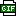此主题相关图片如下：5楼 个性首页 | 信息 | 搜索 | 邮箱 | 主页 | UC加好友发短信假定宇宙的斥力膨胀是线性膨胀，则有                r0  =  m r            和              a0 = m a                                                  (8)其中 m  是膨胀系数，r0 是膨胀前天体的位置，r 是膨胀后天体的位置。    虽然膨胀是非线性的，但是可以用线性膨胀作为它的近似，这样给计算带来很大的方便，而又不影响问题的结论。6楼 个性首页 | 信息 | 搜索 | 邮箱 | 主页 | UC加好友发短信Matlab计算最外层（球面）Post By：2005/9/16 20:16:00

 记号：x 代表宇宙膨胀系数μ____________________________________________________________________________函数文件1 ： aqm.m  （最外层的半径a , 球面qm）function a=aqm(x,H,M)global H Ma=27.8592*(M*(1/x^3-3/x+2)/H^2)^(1/3)____________________________________________________________________________函数文件2 ：  （文件名为zvx1.m ，是速度红移的一次项）function zv1=zvx1(x,H,M)global H Mzv1=0.092928258*(H*M*(1/x^3-3/x+2))^(1/3)____________________________________________________________________________函数文件3 ： zvx2.m  （文件名为zvx2.m ，是速度红移的二次项，即相对论修正项）function zv2=zvx2(x,H,M)global H Mzv2=0.5*（0.092928258*(H*M*(1/x^3-3/x+2))^(1/3)）^2____________________________________________________________________________函数文件4 ：zvx12  （文件名为zvx2.m ，是速度红移的一次项与二次项之和）function zv12=zvx12(x,H,M)global H Mzv12=zvx1(x)+zvx2(x)____________________________________________________________________________函数文件5 ： zex.m  （斥力红移）function ze=zex(x,H,M)global H Mk=0.008635661;ze=0.5*k*(H*M)^(2/3)*(1/x^3-1)/((1/x^3-3/x+2)^(1/3)-k*(H*M)^(2/3)*(0.5/x^3+1))____________________________________________________________________________函数文件6 ：  zvex.m  （速度红移与斥力红移之和）function zve=zvex(x,H,M)global H Mzve=zvx12(x)+zex(x)____________________________________________________________________________函数文件7 ： zqm.m  （球面红移的非线性方程，即：  速度红移 + 斥力红移 - 2.3 = 0 ）function x=zqm(u,H,M)global H Mx=((0.092828258*(H*M*(1/u^3-3/u+2))^(1/3))+0.5*(0.092828258*(H*M*(1/u^3-3/u+2))^(1/3))^2+(0.5*0.008636*(H*M)^(2/3)*(1/u^3-1))/(1/u^3-3/u+2)^(1/3)/(1-0.008636*(H*M)^(2/3)*(0.5/u^3+1)/(1/u^3-3/u+2)^(1/3)))-2.30____________________________________________________________________________函数文件8 ： p.m  （用字母p代表宇宙密度ρ）function p=p(a,M)global Mp=81.2568*M/a^3____________________________________________________________________________函数文件9 ： v0xa.m  （用字母V代表希腊字母Λ，v0即Λ0）function v0=v0xa(x,a)v0=22.70933/x^3/a^3______________________________________________________________________________函数文件10 ：vxa  （用字母V代表宇宙常数Λ）function V=vxa(x,a)V=22.7093347*(1/x^3-1)/a^3______________________________________________________________________________M_文件：  qm.mglobal H M      % 设置 H、M 为全局变量H=input('H=');    % 键盘输入HM=input('M=');u=input('u=');    % u=0.4 是μ的近似值，这里用u代替μx=fzero('zqm',u)  % 解非线性方程，求宇宙膨胀系数μx=input('x=');      % 键盘输入xa=aqm(x)        % 求宇宙半径zvex(x)          % 求速度红移和斥力红移之和v0xa(x,a)        % 求斥力常数Λ0vxa(x,a)          % 求宇宙常数Λp(a,M)          % 求宇宙密度ρ7楼 个性首页 | 信息 | 搜索 | 邮箱 | 主页 | UC加好友发短信Z - r 关系Post By：2005/9/16 20:19:00

 当 Z ＜2.3 时，斥力红移用球内公式（39）计算，速度红移用公式（38）计算，总红移是公式（28）。    当 Z ＞2.3 时，斥力红移用球内公式（40）计算，速度红移用公式（38）计算，总红移是公式（28）。8楼 个性首页 | 信息 | 搜索 | 邮箱 | 主页 | UC加好友发短信Matlab球外的计算Post By：2005/9/16 20:20:00

 记号：w-球外；r-距离或半径；v-速度红移；e-斥力红移；*乘号函数文件 1 ：zvr1.mfunction zv1=zvr1(r,H)global Hzv1=0.003335641*H*r______________________________________________________________________函数文件 2 ：zvr2.mfunction zv2=zvr2(r,H)global Hzv2=0.5*(0.003335641*H*r)^2______________________________________________________________________函数文件 3 ：zvr12.mfunction zv12=zvr12(r,H)global Hzv12=zvr1(r)+zvr2(r)______________________________________________________________________函数文件 4 ：zerw.mfunction ze=zerw(r,M,a,V0)global H M a V0k1=0.005296998;k2=0.240582497;k3=0.360873745;ze=(k1*V0*M*r*r+k2*M/r-k3*M/a)/(1-k1*V0*M*r*r-k2/r)______________________________________________________________________函数文件 5 ：zvew.mfunction z=zvew(r,H,M,a,V,V0)global H M a V V0z=zvr12(r)+zerw(r)______________________________________________________________________M文件 ：qw.mglobal H M a V V0H=68;M=1.0;a=3.59;V=7.039093903;V0=7.528217346;r=input('r=')zvew(r)9楼 个性首页 | 信息 | 搜索 | 邮箱 | 主页 | UC加好友发短信最外层的计算结果列入表2中（附录11），其中 Mv 是与最佳拟合线相符的数值（图7）。为了缩小选择的范围，提出几个限制。    1）在星系的中心区域，维里质量是光学质量的7―10倍，所以                              1．4  5 。这是根据当前发现高红移类星体的趋势的一种判断，从以后的计算结果可以验证这个假设。 根据这些限制条件，我们得到了表3。从表2和表3可以看出宇宙参数的合理范围是                                   H = 65 ―70                          M = 0.9― 1.2                                    a =3.5―3.7                            r = 1.6―2.0 根据自已的观点可以任意选取一组。我选定酌一组是：                                   M =1.0            r =1.75          H =68                                    a = 3.59        m=0.4     表１中的计算结果是采用下列数值进行计算的：                           M=1.0                      H=68                  r =1.750144098                            a =3.594142145                                  m=0.402014863 ――――――――――――――――――――――  *  《天文学与哲学》(论文集)，第271页，中国社会科学出版社，1984年。  **  H.L.西普门，《黑洞》、类星体和宇宙》，第341页，科学出版，1987年。      中国大百科全书《天文学》，第108页，大百科全书出版社，1980年。10楼 个性首页 | 信息 | 搜索 | 邮箱 | 主页 | UC加好友发短信在 Z-m 图中，看出类星体的视星等的下限约为21m.0 。    对于遥远天体，距离和退离速度都使它的亮度减弱。如果退离速度接近光速，那么距离的远近可能成为次要的，而退离速度和退离加速度足以使它的亮度趋于零。    现在的仪器可以观测到28等星。由此可见，类星体视星等的下限标志着它的退离速度不接近光速。

 总数 39 1 2 3 4 下一页

# 天文知识（专业） 一组宇宙计算公式原理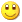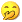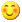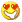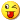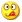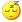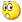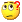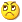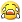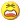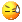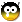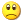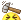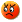签名： 不显示 显示